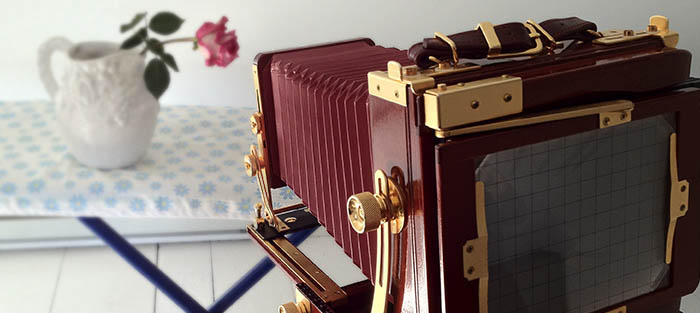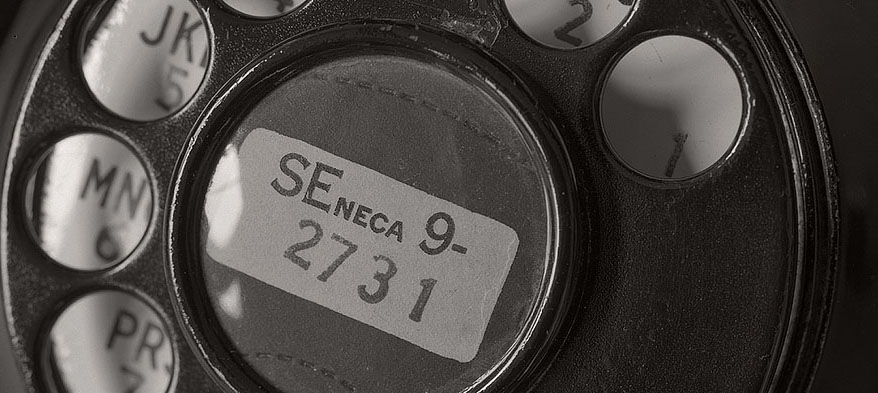Kenneth Morris Lee
Formulas for Bellows Extension and CompensationHow Close Can We Get ?

Given a 200mm lens and 315mm of bellows draw, how close can we get ?

1/F = 1/D + 1/B
where F = focal length, D = distance, B = Bellows Draw

F = 200mm, B = 315mm

1/200 = 1/D + 1/315
1/D = 1/200 - 1/315
1/D = 0.005 - 0.003174603174603
1/D = 0.001825396825397
Distance = 547mm

How Much Bellows Extension Do We Need ?

Given a 360mm lens and 1500mm distance how much bellows draw is required ?

1/F = 1/D + 1/B
where F = focal length, D = distance, B = Bellows Draw

F = 360mm, D = 1500mm

1/360 = 1/1500 + 1/B
1/B = 1/360 - 1/1500
1/B = 0.0027 - 0.0007
1/B = 0.002
Bellows Draw = 500mm

How Much Bellows Extension Do We Need ?

Given a 210mm lens where the desired magnification is 1:3, how much bellows extension is required ?

M = (B-F) / F
where M = magnification ratio, B = bellows extension, F = focal length

M = 1/3, F = 210mm

1/3 = (B-210) / 210
1/3 * 210 = B - 210
70 = B - 210
Bellows Extension = 280mm

How Much Magnification Can We Get ?

Given a 200mm lens and 320mm bellows draw, how much magnification can we get ?

M = (B-F) / F
where M = magnification ratio, B = bellows extension, F = focal length

M = (320-200) / 200
M = 120/200
Magnification = 60%

Given a 150mm lens and 320mm of bellows draw, how much magnification can we get ?

M = (320-150)/150
M = 170/150
Magnification = 1.33 : 1 or 133%

How Much Magnification Do We Get ?

Given a 300mm lens and a distance of 10 feet, how much magnification do we get ?

M = F / (D-F)
where M = magnification ratio, D = distance, F = focal length

F = 300mm, D = 10ft = 3048mm

M = 300 / (3048-300)
M= 300 / 2748
= 10.9 %

Given a 450mm lens and a distance of 15 feet, how much magnification do we get ?

F = 450mm, D = 15ft = 4572mm

M = 450 / (4572-450)
M= 450 / 4122
= 10.9 %

How Much More Exposure Time Is Required ?

Given a 300mm lens, 450mm of bellows draw and a 2-second exposure, how long should the exposure be ?

Compensation = (B / F) squared
where B = Bellows extension and F = Focal length

Compensation = (450/300) ^2
Compensation = 1.5 ^2 = 2.25

Adjusted exposure time is 2 x 2.25 seconds, or 4.5 seconds

How Many More f/stops are Required ?

Given a 150mm lens, 300mm of bellows draw and a 2-second exposure, by how many f/stops should we adjust the exposure ?

Compensation = (((B / F)^2) log2) stops
where B = Bellows extension and F = Focal length

Compensation = ((300 / 150)^2) log2 stops
Compensation = (2^2) log2 stops
Compensation = 4 log2 stops
Compensation = 2 stopsVintage telephone at 1:1 magnification, 150mm lens on 4x5 film.